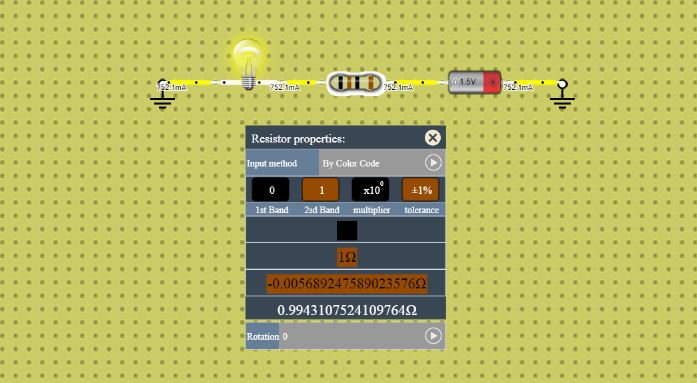# How to use Resistor in lab

Follow the simple steps below in order to use the resistance in your circuit as per your application.

## Steps to use Resistance in Lab

Step 1: Navigate through the list of the devices/components in the above and locate the symbol of Resistance as shown in the snapshot below.Step 2: Click on the icon of the Resistance as shown in the screenshot above or grab the icon, a resistance will appear on the below board.Step 3: Connect the resistance in the circuit and set its value as per your application.### Controlling the properties of the Resistor

When you are working with a circuit click on the resistor and a Gear shape will be displayed near to the resistance as shown in the image below.Click on the gear icon shown in the above screenshot and a box with resistor property will show up as shown in the screen shot below.There is an option in the properties labeled as “Input Method” here are two options to select. You can select either options from “By color Code” or “By text“.

#### Resistance property by Color Code1. The input method is the same as what explained before, in the screenshot above the input method is set to “by color code“.
2. In this section the four color of the resistance is shown with the corresponding value (explained in below screenshots).
3. The Maximum and Minimum value of the resistance with the (+ and -) errors are represented with the resistance value below them.
4. In this the resistance can be rotated at its point in the circuit, the resistance can be rotated  from 0° to 90°, 180° and 270°.In the above screenshot the color with its corresponding value for the first as well as second band is shown which has the range from 0 to 9.In the above screenshot the color and its corresponding value for the multiplier part of the resistance is shown. The range of the multiplier part is from 10^-2 to 10^9.In the above screenshot the color and its corresponding value for the tolerance of the resistance is shown. The range of the tolerance is from (+ & -) 1% to (+ & -) 20%.

#### Resistance property by Text1. The input method is the same as what explained before, in the screenshot above the input method is set to “by text“.
2. In this you can directly enter the value of the resistor and select the range or the order value of the resistance.
3. In this you can set the value of a resistance by scrolling the scrollbar.
4. In this you can control the small fraction of the resistance value in the range of milli, µ (micro) and nano ohms.
5. In this the resistance can be rotated at its point in the circuit, the resistance can be rotated  from 0° to 90°, 180° and 270°.

#### Connecting Resistances in parallelIn the above snapshot shown the two resistances of 1 Ω and 3Ω are connected in parallel. The Battery is connected as a power source which have 1.5V and 2.007A of voltage and current respectively.

We know that the voltage is the same across the parallel loads/devices, hence both the resistors would be having same voltage of 1.5V. However the current is divided in between the resistors as per their value.

We know that the, I=V/R

where, I = current, V = voltage and R = Resistance

with the help of the above expression we can calculate the current across the two 1 Ω and 3Ω resistors.

Current across 1 Ω resistor = 1.5/1 = 1.5A

Current across 3Ω resistor = 1.5/3 = 0.5A

According to the Kirchhoff’s Current law at the junction where 1 Ω and 3Ω resistances are connected in parallel the sum of the Current across 1 Ω resistor and the Current across 3 Ω resistor has to be equal to the current driven by the voltage source.

I (Source Current) = (Current across 1 Ω resistor + Current across 3 Ω resistor)

I = 1.5 + 0.5 = 2A (which is equal to the source current in actual)

#### Resistances with different Error FactorsIn the above snapshot the four resistances A, B, C and D are shown in which the resistances A, B and C have been selected to be have error factor of 2%, 5% and 10% respectively, the resistance property i.e Input method of D is set to “by text”.

The error factor is denoted by the fourth color strip of the resistor, with the error factor the more practical value of resistance is known. The error factor is effected by various factors such as component used to make the resistor, amount of solder used and many other factors.

As shown in the screen shot above we can get a basic idea that the less error factor we have (which is near to zero) the more precise value of the resistance in practical we will get.

The D represents the ideal condition in which the resistance is of exact value which we need in our application.

Note : The D condition in which the resistance has an ideal value does not exist in real life. We can use this feature in the lab in order to check the accuracy of our circuit.

•
•
•
•
•
•